WEBINAR
Numerical Analysis of Magnetic Pulse Welding Process
Thursday, October 5, 2023
Time
SESSION 1
SESSION 2
CEST (GMT +2)
03:00 PM
08:00 PM
EDT (GMT -4)
09:00 AM
02:00 PM
HOME / Applications / Lorentz force computation of a low voltage busbars system under a short-circuit current

# Lorentz force computation of a low voltage busbars system under a short-circuit current

Used Tools:## Problem description

Busbar (Figure 1), composed of a single or a group of conductors, is used as an electrical junction between incoming and outgoing electrical currents.  Some typical applications of these devices can be to form the interconnectedness of the incoming and outgoing electrical transmission lines and transformers at an electrical substation; supplying huge amounts of amperes to the electrolytic process in an aluminum smelter by using large bus bars and interconnecting generators to the main transformers in a power plant.

They are made usually of copper or aluminum in tubular or rectangular or flat shape depending on their application and the amount of currents will be transmitted.  The issues that need to be addressed in the design of busbar systems include temperature rise due to energy losses, energy efficiency and lifetime cost, short-circuit current stresses and protection, and maintenance, etc.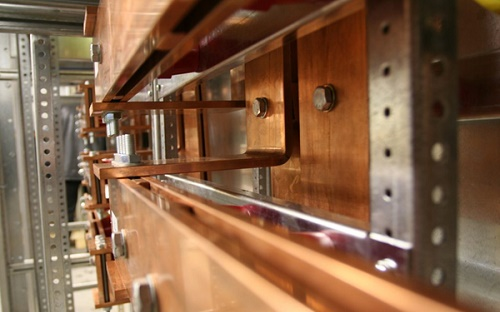Figure 1 - Busbars system 

In this article, the Lorentz force of a low voltage busbars system during a short-circuit regime will be computed by EMS and compared with analytical solution. A system of 3 phase busbars is considered for this analysis . Below is a 3D CAD model of the simulated system built inside SOLIDWORKS (all dimensions are in millimeters).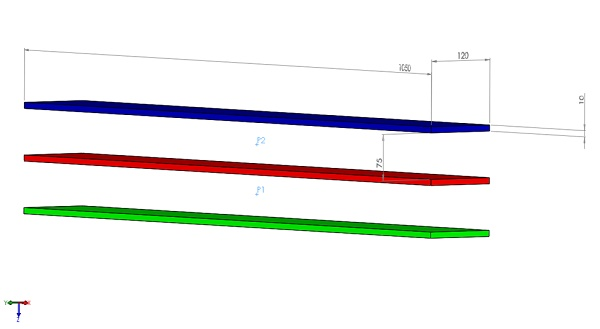Figure 2 - The simulated model

## Transient electromagnetic simulation with EMS

To Study the response of the busbars system during the short-circuit regime, a time-varying analysis is needed. The Transient module of EMS is used to simulate different types of excitation (sinusoidal, pulse, exponential, DC, ...) and generates results in time-domain of magnetic flux, eddy currents, back emf, electromagnetic losses (core loss, eddy loss, hysteresis loss, etc), electromagnetic forces and torques, etc. The studied example will be analyzed using the Transient module of EMS.

Lorentz force and magnetic flux density at each conductor of the busbars system will be computed and compared to ref  results.
To perform an analysis inside EMS after creating a new study, three important steps shall always be followed:

1- Apply the proper materials for all solid bodies
As aforementioned, the three busbars are made of copper due to its higher electrical conductivity.
2- Apply the necessary boundary conditions.

Each conductor, which corresponds to one phase, is defined as a solid coil. Figure 3 contains the short-circuit current circulating at each phase of the busbars system.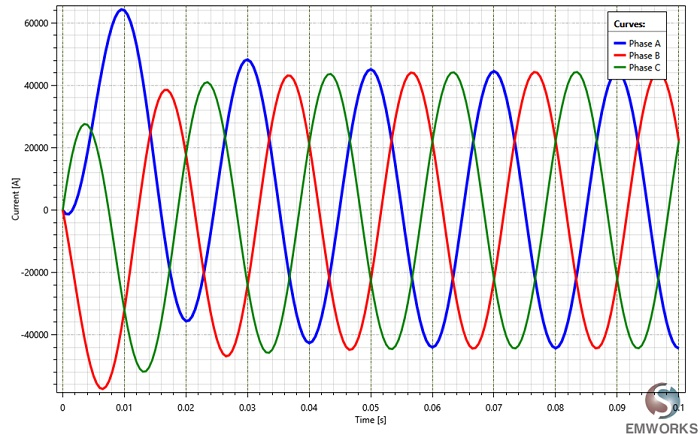Figure 3 - Input currents

3- Mesh and run the model

In the FEM simulation, the meshing step has an important impact in the results accuracy and the computation time. EMS meshes the simulated geometry with tetrahedral elements. The total elements number depends on the dimensions, the shape of the model geometry. EMS allows to control the mesh size on specific bodies or surfaces to enhance the accuracy of the results in these zones. Below is the meshed model. A mesh control of 5 mm was applied on all the conductor bodies.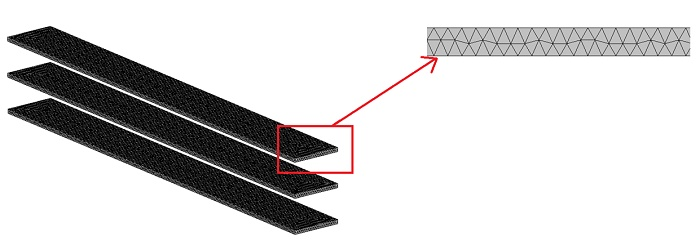Figure 4 - The meshed model

After meshing the model, the simulation step has been proceeded. The analysis duration was set to 0.1s with a time step of 0.0005s.

## Simulation Results

After successfully running the simulation, 3D plots versus time of the magnetic flux, the magnetic field intensity, the applied and eddy currents density, force density and losses density are computed and generated by EMS.
Lamped quantities are listed in EMS Results Table.

Figure 5a) and 5b) show the magnetic flux density distribution in the busbars system at t=0.061s. It is characterized by high spots in the edges of the conductors due to the end effect phenomenon.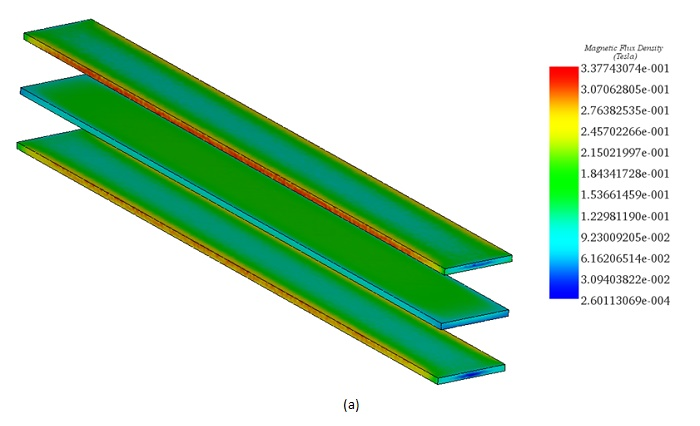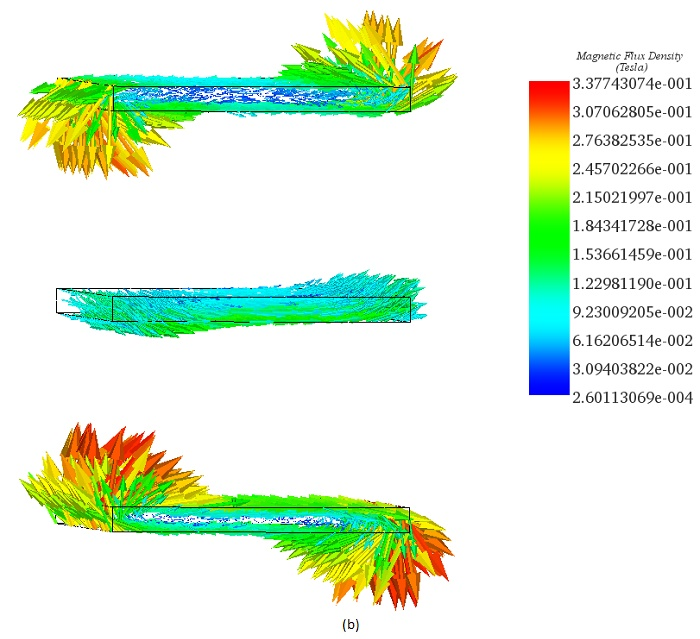Figure 5 - 3D plot of the magnetic flux density: a) Fringe plot, b) Vector plot

Figure 6a) and 6b) contain the plot respectively, of (By) component and the magnitude of the magnetic flux at P1 and P2 (see Figure 2) . As can be concluded from the figures below, the magnetic flux density is mainly in the Y direction and its magnitude is oscillating between 0 and 0.22T.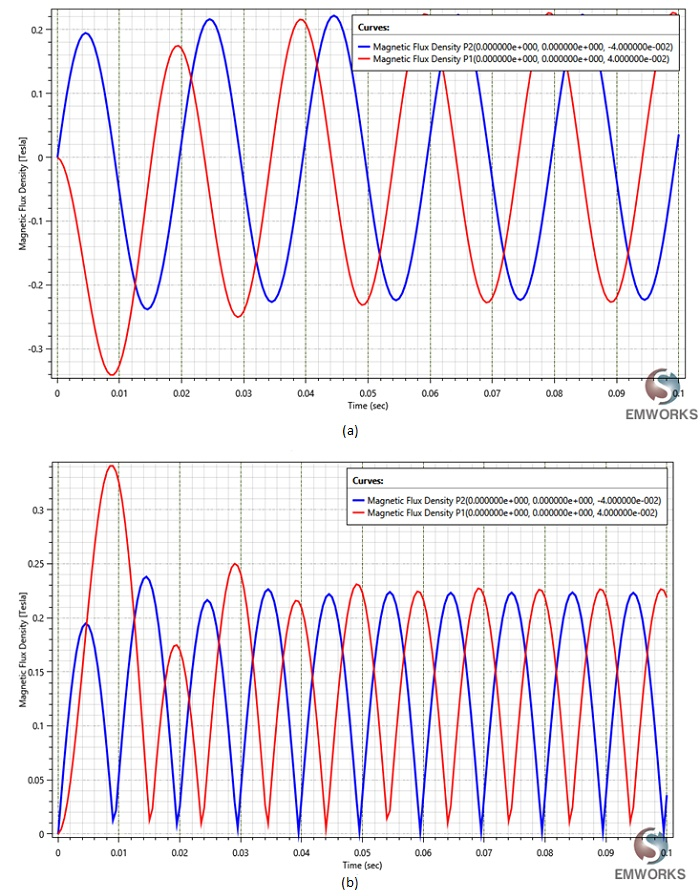Figure 6 - 2D plot at two points P1 and P2 of: a) (By) component of the magnetic flux density, b) The magnitude of the magnetic flux density

Figures 7 and 8 show respectively, the current density distribution (eddy and applied currents) and the volume Ohmic losses in the busbars system at t=0.038s. The zones with the highest losses correspond of the zones with the highest currents.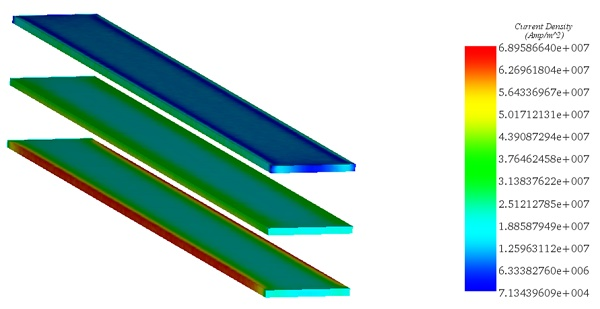Figure 7 - Current density distribution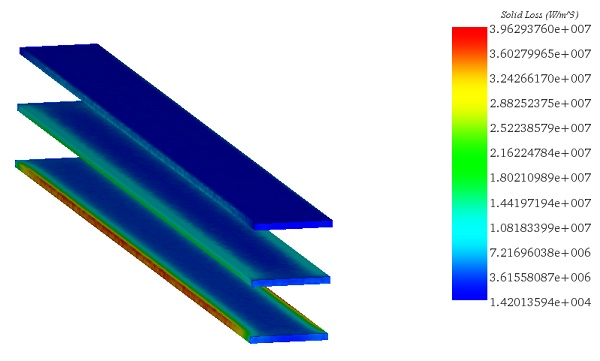Figure 8 - Solid loss distribution

Figure 9 shows the Lorentz forces of each conductor of the busbars system versus time after entering in the short-circuit phase. The Lorentz forces all are in the Z direction and the phase B conductor experiences the highest electromagnetic force.

Table 1 establishes a comparison of the Lorentz force results given by EMS, analytical and ref . The peak values of the force are chosen from the curve of each phase force (skipping the first oscillation) as it is highlighted in the Figure 9. Figure 10 demonstrates the sum of the three forces of each conductor. The curve is oscillating from -10 N to 60 N.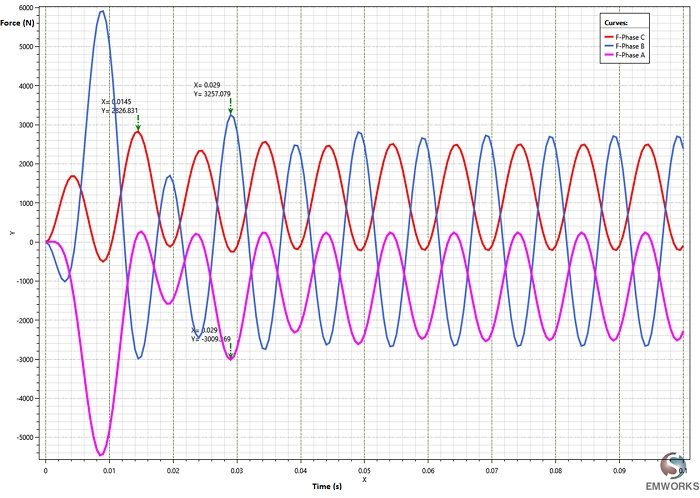Figure 9 - Lorentz force of each conductor phase

Table 1 - Lorentz forces comparison
 EMS Analytical _Ref  Simulation _ Ref  Error (EMS-Analytical) % F_Phase A 3009.37 2945.07 2816.28 2.18 F_Phase B 3257.08 3135.13 3015.44 3.88 F_Phase C 2826.83 2945.07 2816.28 4.01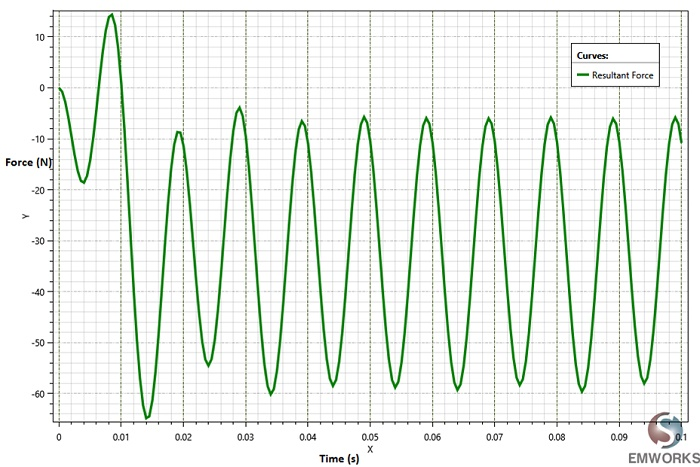Figure 10 - Sum of the three Lorentz forces

## Conclusion

In this example, a busbars system was studied under short-circuit regime. The high amount of current generated during a short-circuit creates high electromagnetic forces which can cause many types of defects on the busbars system.  Estimating the Lorentz forces created by these currents can help to design a system that supports extreme conditions. The force results computed by EMS correlate very well with analytical solution. EMS helps to study busbars systems in both time-varying domain and frequency-domain. Coupling to thermal and structural analysis, EMS allows to predict respectively, the Joule heating and the deformation caused by this Joule heating and/or electromagnetic forces.

#### References

: https://electropak.net/blog/manufacturing/benefits-and-uses-busbars/
: Gholamreza Kadkhodaei, Keyhan Sheshyekani , Mohsen Hamzeh.Coupled electric–magnetic–thermal– mechanical modelling of busbars under short-circuit conditions. The Institution of Engineering and Technology, 2016.